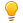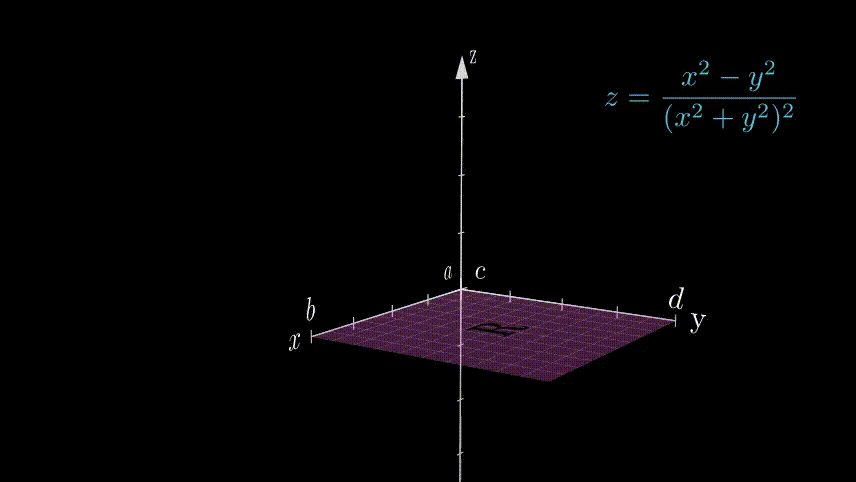# Definition

For double integral of a function $f(x,y)$ in a rectangular region $R=[a, b]\times [c, d]$  we can write,    $$\int_R f(x,y)\ dxdy = \int_a^b\left(\int_c^d f(x,y)\ dx\right)dy = \int_c^d\left(\int_a^b f(x,y)\ dy\right)dx$$
if $\iint_R |f(x,y)|\ dxdy < \infty$, otherwise the integral value is infinite.

Extension for multiple integrals,

If $f$ is a function integrable on $R^n$ and $\int_{R^n}|f\ |dx^n < \infty$  then a multiple integral can be done by dividing it into single integrals (iterated integrals), $$\int_{R^n}f(x_1,x_2\cdots x_n )dx^n= \int\left(\cdots \left(\int f(x_1\cdots x_n )dx_1\right)\cdots \right)dx^n$$
Here we can do $x_1\cdots x_n$ integration in any order if the subsequent integrands become integrable. While we integrate over one variable the others act as constants.

-1

# Motivation

We integrate a function of a single variable easily but how do we integrate functions of more than one variable? We have Riemann Sum method to get the numerical value of any integral if the region is well defined, but for higher dimensions, it has limitations/difficulties. So for doing a multiple integral by hand we have no other way than dividing it into single variable integrals and do them recursively (iterated integrals) by means of some methods and anti-derivative formulas.
Now if we have an integral like $$\int_0^1\int_0^1\dfrac{x^2-y^2}{(x^2+y^2)^2} dxdy$$

It gives two different answers($-\frac{\pi}4$ and $\frac{\pi}4$) for two different order of iteration(first $x$ or first $y$ integration). So it is not obvious that the iteration method gives us the right answer always!
Fubini's theorem guarantees that we will get the same valid answer irrespective of the order of iteration.

In the current topic, we will see how to check if an integral can be done by the method of iteration; we will also see how to take advantage of different orders of iteration.

-1

# Bird's Eye View

Fubini's Theorem gives the conditions under which it is possible to compute a integral as an iterated integral. Double integral is the "smallest" multiple integral where we can use this theorem. If we have a double integral as $\int_a^b\int_c^d f(x,y)\ dxdy$ over a rectangular region $R=[a, b]\times [c, d]$ then we can write,
\begin{align} \int_a^b\int_c^d f(x,y)\ dxdy = & \int_a^b\left(\int_c^d f(x,y)\ dx\right)dy \\
= &\int_c^d\left(\int_a^b f(x,y)\ dy\right)dx\end{align}
If the integral  $\int_a^b\int_c^d |f(x,y)|\ dxdy$ is not finite then the two iterated integral above can give different values and the  iteration method fails.

If the above equalities are valid, we can either do the $x$ integral or the $y$ integral first, but that may not be always possible!
It may happen that $h(x)=\int_c^d f(x,y)\ dy$ is not $x$ integrable  but $g(y)=\int_a^b f(x,y)\ dx$ is $y$ integrable , so in this case we can only integrate over $x$ first and then over $y$.Remarks: The integration limits for different iterations (i.e. first $x$ or first $y$) in the previous example remain unchanged as the integration was over a rectangular region. But for general regions, we have to set the limits as required in the problem for different orders of iteration.

0

# Context of the Definition

In general, we can't do multiple integrations by iterations until some conditions are fulfilled. Fubini's theorem gives us these conditions[1,5],

• If integral of the absolute value is finite($\int_{R^n} |f(x_1\cdots x_n)|\ dx^n \lt \infty$) then we can change the order of integration  in iterated integrals getting the same value always. The assumption that the integral of the absolute value of the function is finite is called "Lebesgue integrability".
• If $\int_{R^n} |f(x_1\cdots x_n)|\ dx^n = \infty$ , different orders of iterated integral may give different values. The reverse of the statement is also true.

Now we see an example where changing the order of integration gives different values--
$$\int_0^1\int_0^1\dfrac{x^2-y^2}{(x^2+y^2)^2} dxdy$$

Method 1 : First we do the $x$ integration ,

\begin{align} \int_0^1 \dfrac{x^2-y^2}{(x^2+y^2)^2} dx &=\int_0^1 \dfrac{-(x^2+y^2)+2x^2}{(x^2+y^2)^2} dx \\
&= \int_0^1 \dfrac{-1}{(x^2+y^2)} dx-\int_0^1 x\dfrac{d}{dx}\left(\dfrac{1}{(x^2+y^2)}\right) dx\\
&= -\int_0^1 \dfrac{1}{(x^2+y^2)} dx- \left[\dfrac{x}{(x^2+y^2)}\right]_0^1+\int_0^1 \dfrac{1}{(x^2+y^2)} dx\\
&= -\dfrac{1}{1+y^2} \\
\text{Now doing the $y$ integration,  }\\ \int_0^1-\dfrac{1}{1+y^2} dy&=-\dfrac{\pi}{4}
\end{align}

Method 2 : First we do the $y$ integration,

\begin{align} \int_0^1 \dfrac{x^2-y^2}{(x^2+y^2)^2} dy &=\int_0^1 \dfrac{(x^2+y^2)-2y^2}{(x^2+y^2)^2} dy \\
&= \int_0^1 \dfrac{1}{(x^2+y^2)} dy+\int_0^1 y\dfrac{d}{dy}\left(\dfrac{1}{(x^2+y^2)}\right) dy\\
&= \dfrac{1}{1+x^2} \\
\text{Now doing the $x$ integration,  }\\ \int_0^1\dfrac{1}{1+x^2} dx&=\dfrac{\pi}{4}
\end{align}

We see  that in the previous two possible iteration of the given double integral we get different answers. Here fubini's theorem implies that $$\int_0^1\int_0^1\left|\dfrac{x^2-y^2}{(x^2+y^2)^2}\right| dxdy=\Large\infty$$

0

0Animation 1: Nature of the Function $\dfrac{x^2-y^2}{(x^2+y^2)^2}$. The blowing up of the function near the origin gives insight about the calculated results.

### ♦ Fubini's theorem over general regions

If we have to set double integral of the function $f(x,y)=xy$ in closed region $R$ between the curves $y=x^2$ and $y=x$, it would be like--
$$\int\int_R (xy)\ dxdy$$

0

0

Animation 2: The function $z=xy$ and the region of integration $R$

We see that function is a good one i.e. no singularities in the region of integration- no infinities. So we can change the order of integration as we want..
We can do it in any order we want! But we have to be a little cautious about the limits of integration.

##### Method 1

Here we first do the $y$  integration.
$$\int_{y=x^2}^x (xy)\ dy= \left[\dfrac{xy^2}{2}\right]_{x^2}^x=\dfrac{x^3}{2}-\dfrac{x^5}{2}$$
Then the $x$ integration ,
$$\int_0^1\left(\dfrac{x^3}{2}-\dfrac{x^5}{2} \right)dx=\left[\dfrac{x^4}{8}-\dfrac{x^6}{12}\right]_{0}^1=\dfrac 1{24}$$

Someone can say why the limit is not like $\int_{y=x}^{x^2}$. It is not so because if you draw the region you will see $y=x^2$ is closer to $x$ axis so it has to be the lower limit of the integration. But if someone has mistakenly done it, he/she has to multiply with a negative sign everywhere.

##### Method 2

Here we first do the $x$  integration. Observe the limits of integration,
$$\int_{x=y}^{\sqrt y} (xy)\ dx= \left[\dfrac{x^2y}{2}\right]_{y}^{\sqrt y}=\dfrac{y^2}{2}-\dfrac{y^3}{2}$$
Then the $y$ integration ,
$$\int_0^1\left(\dfrac{y^2}{2}-\dfrac{y^3}{2} \right)dy=\left[\dfrac{y^3}{6}-\dfrac{y^4}{8}\right]_{0}^1=\dfrac1{24}$$

The two methods give the same result as expected .

0

0

Animation 3: The two methods of iteration for the double integral.

So to conclude we cannot always do a multiple integral by the method of iterations, for doing so we have to "get permission from Fubini(Fubini's theorem)!". After checking the function if he says "I am pleased with the function's behavior in the integration region", then we can do the iterated integral without any fear of invalid answer.

# Applications

1.  Suppose we have a function which satisfies the conditions of Fubini's theorem, then we can change the order of integration as we want. Now the advantage is if the integration is not doable in one way we can try it the other way to get the result. One such integral is $\int\int_R e^{-y^2} dxdy$ in the region between $y$ axis, $x=y$ and $y=1$ line.
•  If we try to do the y integration first $\implies \int_0^1(\int_x^1 e^{-y^2} dy)dx$, we will fail as $e^{-y^2}$ has no nice anti-derivative.
• But if we try the other way we can do it easily. $$\int_0^1\int_0^y e^{-y^2} dx\ dy=\int_0^1\left[xe^{-y^2}\right]_0^ydy=\int_0^1ye^{-y^2}dy\\ =\dfrac12\int_0^1 e^{-y^2}d(y^2)=\left[-\dfrac{e^{-y^2}}{2}\right]_0^1=\dfrac12 (1-e^{-1})$$

2. In a quite similar way, if we have some boundary curve like shown in the below Figure, we can change the order of integration as needed as long as the integral respects the fubini's criteria for iterability.

0

0

Animation 4: Setting limits in a curvy region.

3. To evaluate the value of Gaussian integral- which is one of the most useful and elementary integrals, we use Fubini's theorem.
$$\int_{-\infty}^{\infty}e^{-x^2}dx=\sqrt \pi$$
See Ref: for hints to prove this.

4. Another standard application of Fubini’s theorem is the inversion formula for Fourier transform.

0

# History

The special case of Fubini's theorem was known to Leonhard Euler in the 18th century. In 1904 Henri Lebesgue extended this to bounded measurable functions on a product of intervals. In 1906 Levi proposed an extension of the theorem to functions that were integrable rather than bounded, and this was proved by Fubini in 1907, known as "Fubini's Theorem". In 1909 Leonida Tonelli gave a variation of Fubini's theorem.

Guido Fubini was an Italian mathematician. Fubini was well liked by his colleagues at Princeton and stories about him abound. He once gave a lecture on ballistics in which he showed that if you fired a projectile of a certain shape, then under the right conditions it could double back on itself and hit your own troops. Then, tongue in cheek, he suggested that one could fool the enemy by aiming this “Fubini Gun” at one’s own troops and hit the unsuspecting enemy after the projectile reversed direction.  

Fubini was exceptionally short, which occasionally caused problems. The story goes that one day his worried landlady called his friends to report that he had not come home. After searching everywhere, including the area near the local lake, it was discovered that Fubini was trapped in a stalled elevator and was unable to reach any of the buttons. Fubini celebrated his rescue with a party and later left a sign in his room that said, “To my landlady: When I am not home at 6:30 at night, please check the elevator. . . .”    

0

# Pause and Ponder

Did you find any similarities between partial differentiation and the iteration method in integration?

Fubini's theorem was formulated in 1907 but people have done multiple integrals before that, how? (See Cavalieri's Principle)

Is there any situation where Fubini's theorem fails?

This theorem is rigorously proved by measure theory.

You should know that doing multiple integrals in higher dimensions by hand is most of the times very laborious/utterly difficult, so people prefer to do it by some numerical methods over computers where we don't need Fubini's theorem, one such widely used numerical method is Monte-Carlo method.

0

# References

1.  Hubbard, John  Hamal, Barbara  Burke. “4.3 Fubini's Theorem and Iterated Integrals.” Vector Calculus, Linear Algebra, And Differential Forms -A Unified Approach., Prentice Hall,page-387
2.  Fubini theorem. Encyclopedia of Mathematics. URL: http://encyclopediaofmath.org/index.php?title=Fubini_theorem&oldid=44743
3. https://tutorial.math.lamar.edu/Classes/CalcIII/IteratedIntegrals.aspx
4. https://sites.math.washington.edu/~morrow/335_12/fubini.pdf
5. https://link.springer.com/content/pdf/bbm%3A978-0-85729-380-0%2F1.pdf
6. https://web.ma.utexas.edu/users/m408m/Display15-2-3.shtml
7. Howard Anton, Irl bivens, Stephen Davis."14.1.3 Fubini’s Theorem." Calculus: Early Transcendentals,10th Edition, Wiley Publishers.ISBN 978-0-470-64769-1, page-1004
8. Weisstein, Eric W. "Singularity." From MathWorld--A Wolfram Web Resource. https://mathworld.wolfram.com/Singularity.html
9. https://www.reed.edu/physics/faculty/wheeler/documents/Miscellaneous%20Math/Gaussian%20Integral%20Evaluation.pdf
10. Weisstein, Eric W. "Monte Carlo Method." From MathWorld--A Wolfram Web Resource. https://mathworld.wolfram.com/MonteCarloMethod.html

0

# Further Reading

1. Hubbard, John  Hamal, Barbara  Burke. “4.3 Fubini's Theorem and Iterated Integrals.” Vector Calculus, Linear Algebra, And Differential Forms -A Unified Approach., Prentice Hall,page-387,
2. Billingsley, Patrick (1995), "Product Measure and Fubini's Theorem", Probability and Measure, Wiley, page. 231–240
3. Weir, Alan J. (1973), "Fubini's Theorem", Lebesgue Integration and Measure, Cambridge University Press, page. 83–92
4. Tonelli's theorem : A successor of fubini's theorem
5. Kuratowski-Ulam theorem : category analogue of Fubini's theorem
6. Numerical methods of integration. Monte Carlo Method.

0

 Contributor: Mentor & Editor: Verified by: Approved On:

The following notes and their corrosponding animations were created by the above-mentioned contributor and are freely avilable under CC (by SA) licence. The source code for the said animations is avilable on GitHub and is licenced under the MIT licence.

The work under this website is licenced under a Creative Commons Attribution-Share Alike 4.0 International License CC BY-SA